Problem

# Consider the following frequency tabulation of leaf weights (in grams): Using t...

Consider the following frequency tabulation of leaf weights (in grams):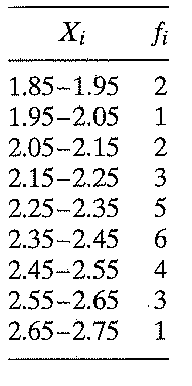Using the midpoints of the indicated ranges of Xi,

(a) Calculate the mean leaf weight using Equation 3.2, and

(b) Calculate the mean leaf weight using Equation 3.3.

(c) Calculate the median leaf weight using Equation 3.4, and

(d) Calculate the median using Equation 3.5.

(e) Determine the mode of the frequency distribution.

Reference quation-3.2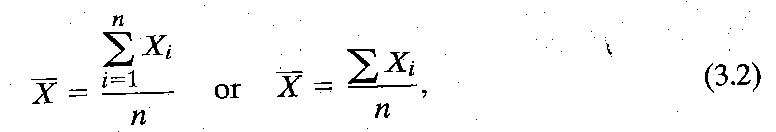Reference quation-3.3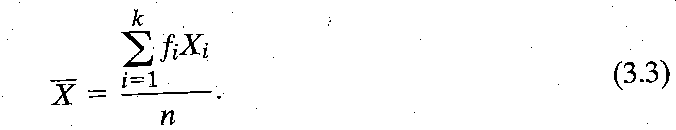Reference quation-3.4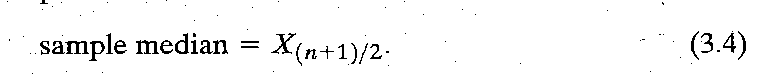Reference quation-3.5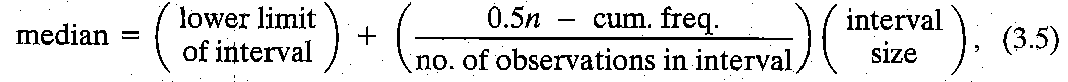#### Step-by-Step Solution

Solution 1

The frequency table of leaf weights is provided to solve the sub parts of the question.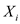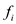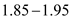2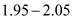1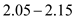2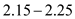3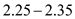5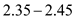6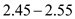4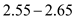3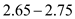1

(a)

The mean leaf weight can be calculated by using equation 3.2 as;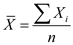From the above provided value the table can be made as;Mid point x Fx2 1.9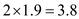1 2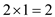2 2.1 4.23 2.2 6.65 2.3 11.56 2.4 14.44 2.5 103 2.6 7.81 2.7 2.7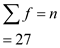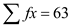So, the mean leaf age can be calculated as: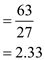Therefore, the mean leaf age is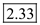g.

(b)

The mean leaf weight can be calculated by using equation $$3.3$$ as;

$$\bar{X}=\frac{\sum f x}{n}$$

Substituting the values from the above table:

\begin{aligned} &=\frac{63}{27} \\ &=2.33 \end{aligned}

(c)

The sample median can be calculated by using equation $$3.4$$ as;

Sample Median $$=X_{(n+1) / 2}$$

From the above calculated table in part (a) the value of $$n$$ is 27 . So, Sample Median will be;

\begin{aligned} &=X_{(n+1) / 2} \\ &=X_{(27+1) / 2} \\ &=X_{28 / 2} \\ &=X_{14} \end{aligned}

The value come by this process is 14 and 14 is most close to $$14.4$$ in the column $$f x$$ from the above table. So, the median will be $$2.4 \mathrm{~g}$$.

(d)

The sample median can be calculated by using equation 3.5 as;

Median = lower limit of interval+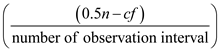interval size

Here, cf is cumulative frequency.

For using the formula firstly calculate the cumulative frequency shown in the table:Mid point x Fx cf2 1.9 3.8 21 2 2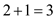2 2.1 4.2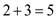3 2.2 6.6 85 2.3 11.5 136 2.4 14.4 194 2.5 10 233 2.6 7.8 261 2.7 2.7 27Substituting the values in above formula: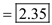So, the median is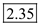g.

(e) Mode: It is highest frequency value in the observation. From the above table values it can see that the highest frequency is 6 in the observation whose corresponding x value is 2.4. Therefore, the mode is 2.4 g.